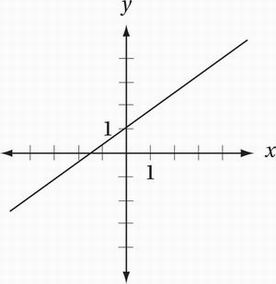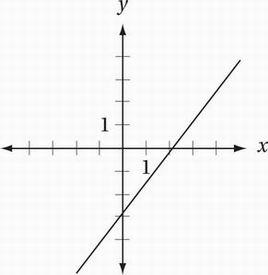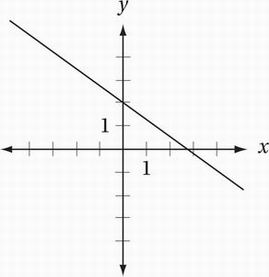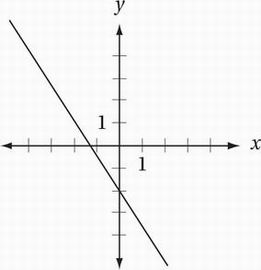# SAT Math Multiple Choice Question 614: Answer and Explanation

### Test Information

Question: 614

14. If k > 2, which of the following could be the graph of y + x = k(x - 1) in the xy-plane?

• A.• B.• C.• D.Explanation:

B

Algebra (graphs of linear equations) HARD

Given equation:

y + x = k(x - 1)

Subtract x:

y = k(x - 1) - x

Distribute:

y = kx - k - x

Collect like terms:

y = (k - 1)x - k

The slope of this line is k - 1 and its y-intercept is - k. If k > 2, then k - 1 > 1, and - k < -2. In other words, the slope of the line is greater than 1 and the y-intercept is less than -2. The only graph with these features is the one in choice (B).